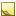﻿TimeInterval data typeTimeInterval data type

The data type TimeInterval represents duration of time or elapsed time that is measured as a positive or negative number of days, hours, minutes, seconds, and fractions of a second.

TDE provides two ways to specify units for TimeInterval data type values:

• Assign units in the Output section of the template
• Assign units in the input dataNote

The TDE data types for quantifiable data (numeric values with units of measure) are all stored as double and are handled similarly for template scripting. When you test equivalency make sure to account for the double format. For example, 1 might actually be stored as 0.999999. Either round off the values being compared, or use ranges for the comparison.

This topic contains the following sections:Assign unit type for TimeInterval values in a template

You can use the following unit type values to assign units to TimeInterval values in the Output section of a template.

Use this format for retrieve templates, and for create and update templates where data inputs do not specify a unit type.

• Second or sec — default

• Minute or min

• Hour or hour

• Day or day

• Month or month

• Year or year

• Nanosecond or ns

• Microsecond or us

• Millisecond or ms

Data from TDE defaults to the first unit listed for each data type. In the [Output] section, you can convert these values to the units you need.

Example: Retrieve template

```[Output]
MinutesUntilArrival(min) = Input.CurrentEstimatedTime   # converts sec to min```

Example

The following example shows the template output for assigning the unit type hours to the TotalEngineTime value from the Unit table.

```[Output]
EngineUsage(hour) = Input.TotalEngineTime```Assign unit type to TimeInterval values in input data

You can use the following values when assigning units to TimeInterval values in the input values for Create and Update templates.

• second, seconds, sec, or secs (default)
• minute, minutes, min, or mins
• hour or hours
• day or days
• month or months
• year or years
• nanosecond, nanoseconds, or ns
• microsecond, microseconds, or us
• millisecond, milliseconds, or ms
• Specify using Valid ISO 8601 time interval format, for example HH:mm:ss.mmm

In the input data, a TimeInterval value can be a positive or negative integer or floating point value. You can also specify multiple units for each input by combining valid units, as shown in the following examples:

Valid TimeInterval Input values
```2 hours
2 hours 10 minutes
2 days
1 year 2 months 3 days 4 hours 5 minutes 6 seconds
04:05:06.789```

Duplicate fields in one interval are not allowed. Also, if the input has an ISO 8601 field, the hours, minutes, seconds, and milliseconds fields are not allowed. For example, if you specify the value -00:01:00 5 hours TDE returns an error because both fields specify a value with hours.

Example

The following example shows the input data to specify TimeInterval units for Shift columns.

```ShiftDuration, MaximumDuration
"08:00:00","10 hours 30 mins"```

You can use the following template to populate the Shift table using the supplied input data.

```RegularShift = Input.ShiftDuration # specify hours in a normal shift
OverTimeShift = Input.MaximumDuration # specify hours permitted for overtime```Field length

The effective range of this data type is: ±9.22 × 1011 secJavaScript conversion

When you use the optional [Script] section to insert your own JavaScript functions, access this data type as follows:

Constructor
JavaScript
`new TimeInterval({Unit:Value})`
Available fields for (get,set)

You can access the valid units of measure as fields:

• .Second

• .Minute

• .Hour

• .Day

• .Month

• .Year

• .Nanosecond

• .Microsecond

• .Millisecond